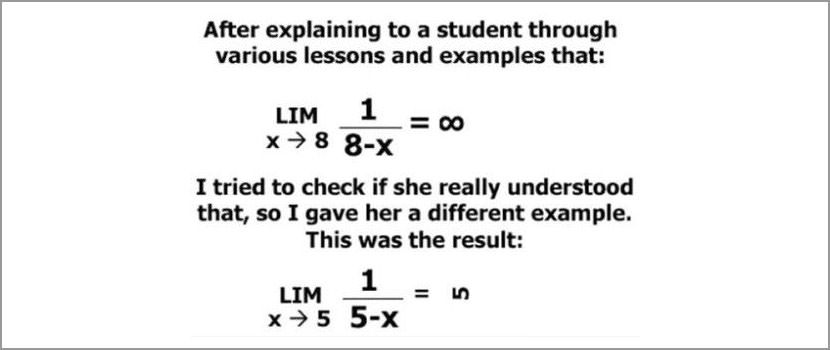## Monday, April 7, 2008

### MathsAlgebraic Formulae

i) Square roots of 4x divided by y-1 = 3, then y=...

ii) x to the power of 6 = y to the power of 2 divided by 81.Express y as the subject of the formulae.

These are the questions confused me. wakaka...Don't want to try to solve them anymore.My head was craking !One of the ways of doing Mathematics question.